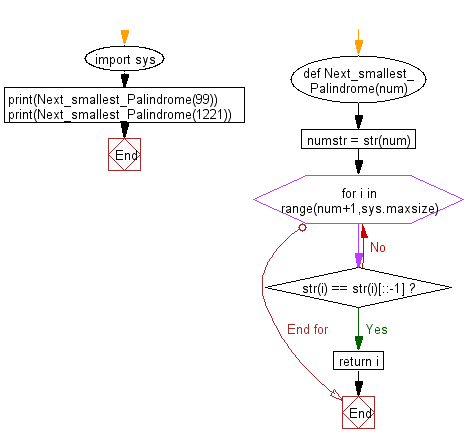﻿ Python Math: Find the next smallest palindrome of a specified number - w3resource# Python Math: Find the next smallest palindrome of a specified number

## Python Math: Exercise-22 with Solution

Write a python program to find the next smallest palindrome of a specified number.

A palindromic number or numeral palindrome is a number that remains the same when its digits are reversed. Like 15951, for example, it is "symmetrical". The term palindromic is derived from palindrome, which refers to a word (such as " REDIVIDER" or even "LIVE EVIL ") whose spelling is unchanged when its letters are reversed.

Sample Solution:-

Python Code:

``````import sys
def Next_smallest_Palindrome(num):
numstr = str(num)
for i in range(num+1,sys.maxsize):
if str(i) == str(i)[::-1]:
return i

print(Next_smallest_Palindrome(99));
print(Next_smallest_Palindrome(1221));
```
```

Sample Output:

```101
1331
```

Pictorial Presentation:Flowchart:Python Code Editor:

Have another way to solve this solution? Contribute your code (and comments) through Disqus.

What is the difficulty level of this exercise?

Test your Programming skills with w3resource's quiz.

﻿

```>>> students = [{'name': 'John', 'score': 98}, {'name': 'Mike', 'score': 94}, {'name': 'Jennifer', 'score': 99}]